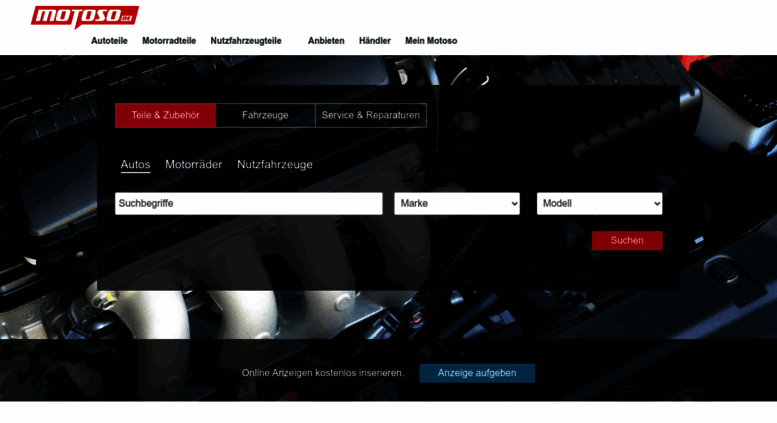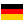motoso.de: Autoteile, Autos, Gebrauchtwagen, Motorräder - motoso.de

Autoteile und Motorradteile, Neu- und Gebrauchtwagen, Motorräder und Tuning-Zubehör - motoso.de ist Dein Auto- und Motorradmarkt im Internet.

• 58/100

Normal result
• 5

Successful tests
• 3

Failed tests
• First response

283 ms

4 sec

• Page rendered

184 ms

4.5 sec

Welcome to motoso.de homepage info - get ready to check Motoso best content for Germany right away, or after learning these important things about motoso.de

We analyzed Motoso.de page load time and found that the first response time was 283 ms and then it took 4.2 sec to load all DOM resources and completely render a web page. This is a poor result, as 60% of websites can load faster.

Page optimization

•  HTML 68.8 kB Images 341.9 kB Javascripts 515.1 kB CSS 191.1 kB

In fact, the total size of Motoso.de main page is 1.1 MB. This result falls beyond the top 1M of websites and identifies a large and not optimized web page that may take ages to load. 40% of websites need less resources to load. Javascripts take 515.1 kB which makes up the majority of the site volume.

• Original 68.8 kB
• After minification 63.0 kB
• After compression 14.4 kB

HTML optimization

HTML content can be minified and compressed by a website’s server. The most efficient way is to compress content using GZIP which reduces data amount travelling through the network between server and browser. HTML code on this page is well minified. It is highly recommended that content of this web page should be compressed using GZIP, as it can save up to 54.4 kB or 79% of the original size.

• Original 341.9 kB
• After optimization 310.9 kB

Image optimization

Image size optimization can help to speed up a website loading time. The chart above shows the difference between the size before and after optimization. Motoso images are well optimized though.

• Original 515.1 kB
• After minification 430.4 kB
• After compression 148.3 kB

JavaScript optimization

It’s better to minify JavaScript in order to improve website performance. The diagram shows the current total size of all JavaScript files against the prospective JavaScript size after its minification and compression. It is highly recommended that all JavaScript files should be compressed and minified as it can save up to 366.8 kB or 71% of the original size.

• Original 191.1 kB
• After minification 155.3 kB
• After compression 29.9 kB

CSS optimization

CSS files minification is very important to reduce a web page rendering time. The faster CSS files can load, the earlier a page can be rendered. Motoso.de needs all CSS files to be minified and compressed as it can save up to 161.2 kB or 84% of the original size.

Network requests diagram

• motoso.de
• www.motoso.de
• motoso_style.jsp
• rc
• scripts.jsp
• jquery.min.js
• jquery-ui.js
• jquery.hoverIntent.minified.js;jsessionid=45C0B17D6CAB1275D74F07843EA448C2.t1
• jquery.cleditor.js;jsessionid=45C0B17D6CAB1275D74F07843EA448C2.t1
• jquery.print.js;jsessionid=45C0B17D6CAB1275D74F07843EA448C2.t1
• jquery-motoso.js;jsessionid=45C0B17D6CAB1275D74F07843EA448C2.t1
• iam.js
• cars_partners_sm.jpg
• logo_mitula_autos.gif
• Startseite;sz=728x90,728x180;kw=;n3=1;n4=3;n5=5;n6=1;n7=4;n8=2;n9=5;n10=5;n11=1;n12=6;n17=4;n18=4;n20=4;n21=4;n23=2;n41=4;n43=4;n46=4;n60=4;n75=1;n76=0;n77=0;n78=1;n79=1;n81=0;n82=0;n83=1;n84=0;n86=1;n88=0;n118=0;n119=0;d9=0;n95=0;n127=0;n66=1;n99=1;n97=1;n101=1;n102=1;n104=1;n105=1;n113=1;n114=1;n115=1;n116=1;n74=2;n98=9;d7=2;d10=3;n128=9;tile=2;dcopt=ist;ord=6073075891472399
• t.js
• defaultImage.gif
• impr
• rtb
• match
• js
• 0
• t.js
• t.js
• analytic.js
• 1.gif
• uid.html
• banner
• pixel
• redirect
• cm.gif
• 2.gif
• callback.js
• impr
• 2.gif
• 135
• 2.gif
• user-registering
• lr.gif
• redirect
• ca-pub-8813347375005258.js
• zrt_lookup.html
• mtrcs_284704.js
• mo_logo1.gif
• index_teaser_car_big.jpg
• mo_icons_vehicles.png
• Startseite;sz=300x250,336x280;kw=;n3=1;n4=3;n5=5;n6=1;n7=4;n8=2;n9=5;n10=5;n11=1;n12=6;n17=4;n18=4;n20=4;n21=4;n23=2;n41=4;n43=4;n46=4;n60=4;n75=1;n76=0;n77=0;n78=1;n79=1;n81=0;n82=0;n83=1;n84=0;n86=1;n88=0;n118=0;n119=0;d9=0;n95=0;n127=0;n66=1;n99=1;n97=1;n101=1;n102=1;n104=1;n105=1;n113=1;n114=1;n115=1;n116=1;n74=2;n98=9;d7=2;d10=3;n128=9;tile=4;ord=6073075891472399
• osd.js
• 1.gif
• analytic.js
• mtrcs_284704.js
• 602
• usersync.gif
• pixel
• generic
• redirect
• 100119041458060110
• 2.gif
• generic
• 2.gif
• cm.gif
• 2.gif
• 2.gif
• 2.gif
• 2.gif
• 2.gif
• 3765016772306774406
• abg.js
• m_js_controller.js
• 2.gif
• s
• redir.html
• push
• 1ddef546-40ff-4ff6-875f-24db911c8dc5.jpg
• mtrcs_284704.js
• Startseite;sz=120x600,160x600,200x600;kw=;n3=1;n4=3;n5=5;n6=1;n7=4;n8=2;n9=5;n10=5;n11=1;n12=6;n17=4;n18=4;n20=4;n21=4;n23=2;n41=4;n43=4;n46=4;n60=4;n75=1;n76=0;n77=0;n78=1;n79=1;n81=0;n82=0;n83=1;n84=0;n86=1;n88=0;n118=0;n119=0;d9=0;n95=0;n127=0;n66=1;n99=1;n97=1;n101=1;n102=1;n104=1;n105=1;n113=1;n114=1;n115=1;n116=1;n74=2;n98=9;d7=2;d10=3;n128=9;tile=5;ord=6073075891472399
• 2.gif
• iframe.html
• redirect
• 2.gif
• 2.gif
• revoke
• submit
• pixel
• 2.gif
• 938
• 7-1I540iwtLQZRDhQUP9L-vt70m6ZeYTcveWqB03r34.js
• 2.gif
• impr
• submit
• rtb
• mtrcs_284704.js
• 1252
• analytics.js
• tx.io
• collect
• pixel
• gum
• revoke
• 15899743288392622299
• push
• tx.io
• pixel
• pixel
• 1945

Our browser made a total of 125 requests to load all elements on the main page. We found that 7% of them (9 requests) were addressed to the original Motoso.de, 22% (28 requests) were made to Dmp.theadex.com and 12% (15 requests) were made to Ih.adscale.de. The less responsive or slowest element that took the longest time to load (718 ms) relates to the external source Assets.motoso.de.

Requests

The browser has sent 102 CSS, Javascripts, AJAX and image requests in order to completely render the main page of Motoso. We recommend that multiple CSS and JavaScript files should be merged into one by each type, as it can help reduce assets requests from 38 to 1 for JavaScripts and as a result speed up the page load time.

38

Javascripts

49

Images

1

CSS

14

AJAX

102

All

Possible request optimization

1

Javascripts

10

Images

1

CSS

14

AJAX

76

Optimized

26

All

Normal result

Redirects

As for redirects, our browser was forwarded to http://www.motoso.de/ before it reached this domain.

Motoso.de uses IP addresses which are currently shared with 2 other domains. The more sites share the same stack of IP addresses, the higher the host server’s workload is. It is strongly recommended that the host server should be changed or the hosting provider should be requested to give a different (separate) IP address for this domain.

Normal result

IP Trace

motoso.de

my.motoso.de

home.motoso.de

18.157.56.170

motoso.de

18.159.30.8

DNS records

Type Host Target/ip TTL Other
A

motoso.de

3.122.66.254 600
NS

motoso.de

nsd6.schlundtech.de 600
NS

motoso.de

nsc6.schlundtech.de 600
NS

motoso.de

nsa6.schlundtech.de 600
NS

motoso.de

nsb6.schlundtech.de 600

Language and encoding

Good result

Language

DEDetected

DEClaimed

Encoding

UTF-8

Claimed

Language claimed in HTML meta tag should match the language actually used on the web page. Otherwise Motoso.de can be misinterpreted by Google and other search engines. Our service has detected that German is used on the page, and it matches the claimed language. Our system also found out that Motoso.de main page’s claimed encoding is utf-8. Use of this encoding format is the best practice as the main page visitors from all over the world won’t have any issues with symbol transcription.

Similarly rated websites

HTTPS certificate

SSL Certificate

Motoso.de has no SSL certificate. Web browsing can be safer with HTTPS connection, so we suggest that it should be obtained for this site.

Poor result

Visitor World Map

Country of origin for 81.9% of all visits is Germany. It lies approximately 4880 miles away from the server location (United States) and such a long distance can negatively affect website speed, as it takes some time for data to travel back and forth between those places. That’s why one of the best ways to speed up Motoso.de page load time for the majority of users is moving the server to Germany or just closer to the user base.

Poor result

Newly analyzed websites

Poor result

Social Sharing Optimization

Open Graph description is not detected on the main page of Motoso. Lack of Open Graph description can be counter-productive for their social media presence, as such a description allows converting a website homepage (or other pages) into good-looking, rich and well-structured posts, when it is being shared on Facebook and other social media. For example, adding the following code snippet into HTML <head> tag will help to represent this web page correctly in social networks:

motoso.de